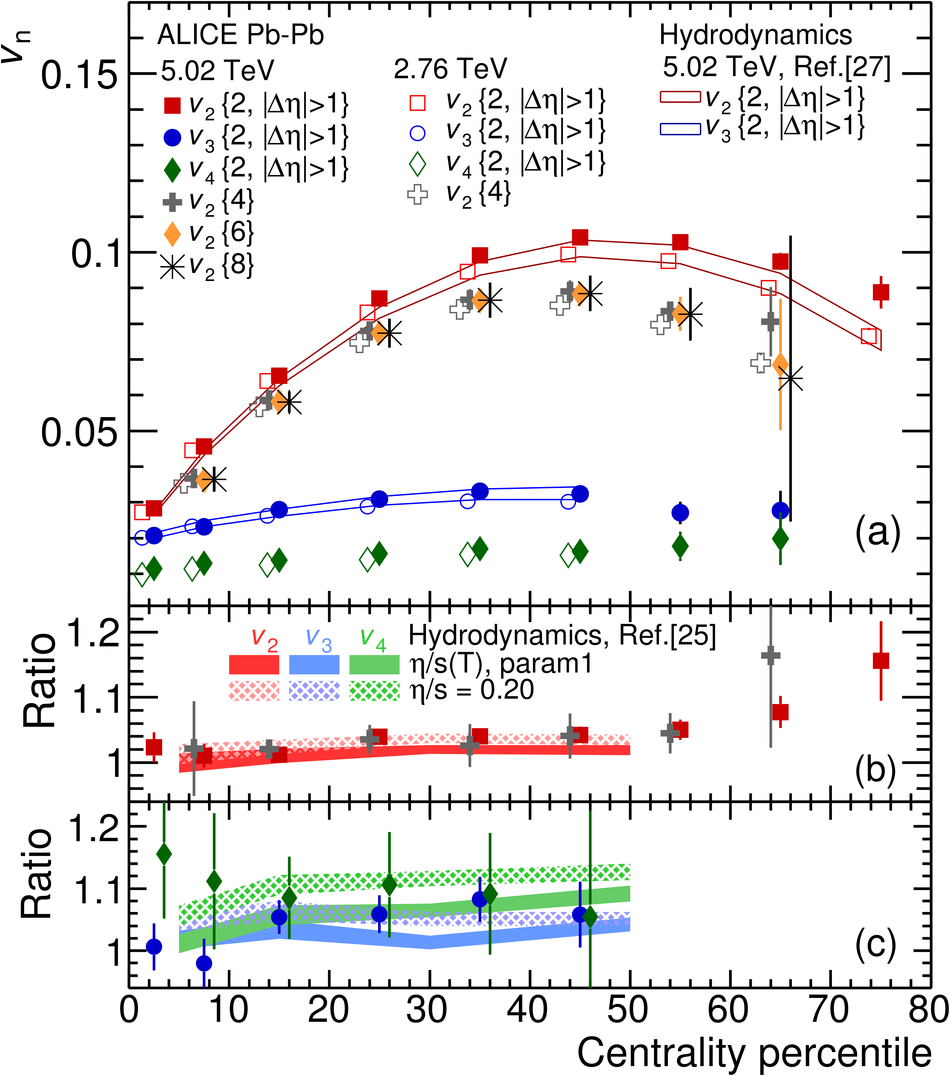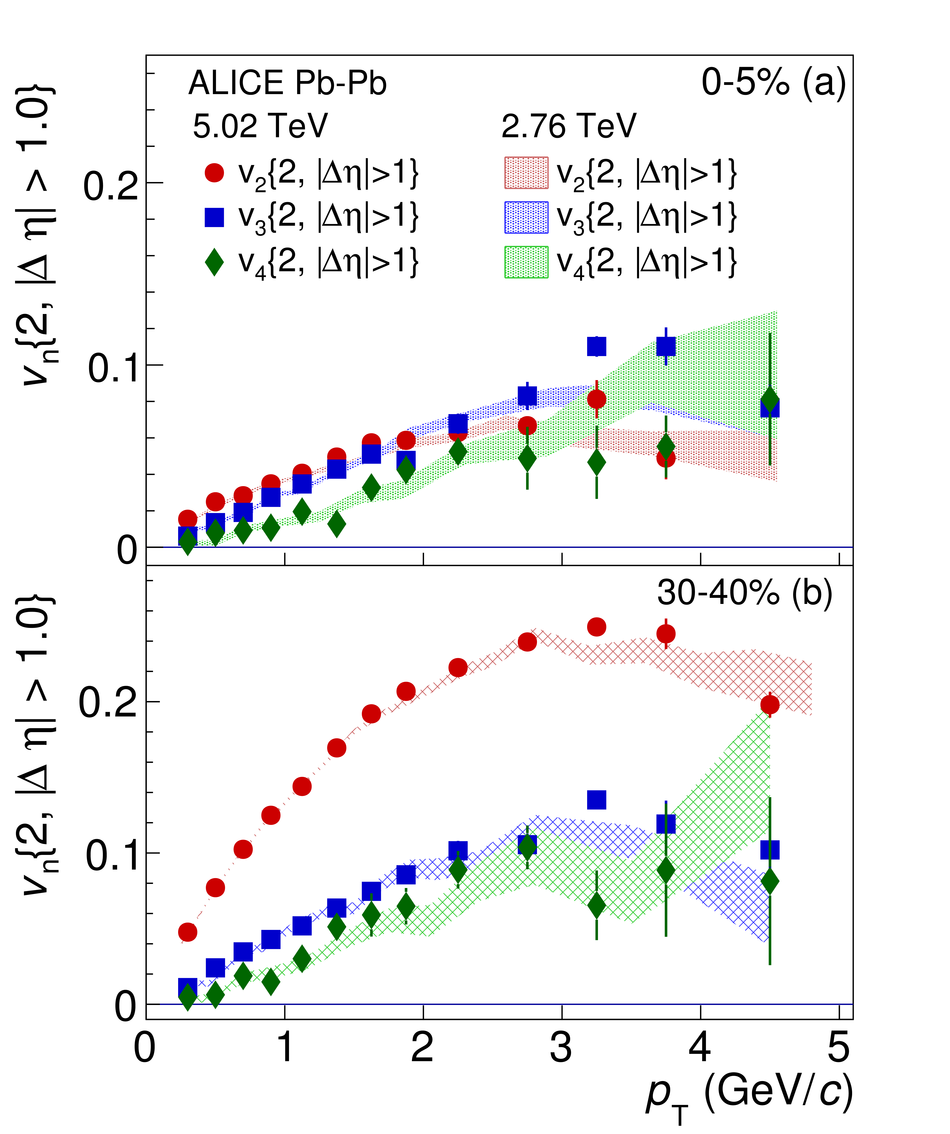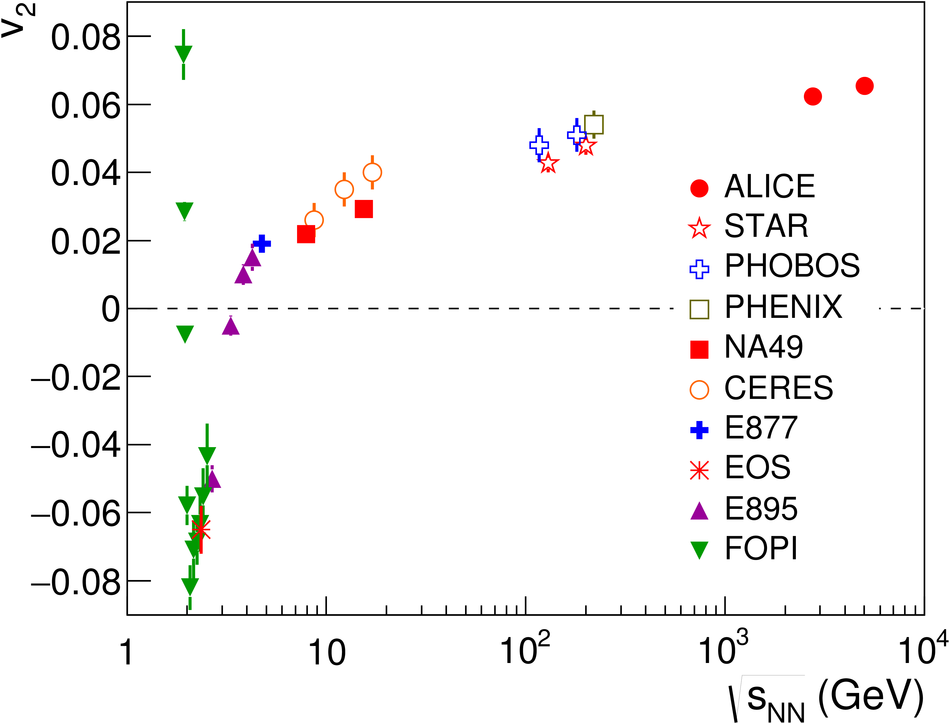Anisotropic flow of charged particles in Pb-Pb collisions at $\sqrt{s_{\rm NN}}=5.02$ TeV

We report the first results of elliptic ($v_2$), triangular ($v_3$) and quadrangular flow ($v_4$) of charged particles in Pb-Pb collisions at $\sqrt{s_{\rm NN}}=5.02$ TeV with the ALICE detector at the CERN Large Hadron Collider. The measurements are performed in the central pseudorapidity region $|\eta|<~0.8$ and for the transverse momentum range $0.2<~p_{\rm T}<~5$ GeV/$c$. The anisotropic flow is measured using two-particle correlations with a pseudorapidity gap greater than one unit and with the multi-particle cumulant method. Compared to results from Pb-Pb collisions at $\sqrt{s_{\rm NN}}=2.76$ TeV, the anisotropic flow coefficients $v_{2}$, $v_{3}$ and $v_{4}$ are found to increase by ($3.0\pm0.6$)%, ($4.3\pm1.4$)% and ($10.2\pm3.8$)%, respectively, in the centrality range 0-50%. This increase can be attributed mostly to an increase of the average transverse momentum between the two energies. The measurements are found to be compatible with hydrodynamic model calculations. This comparison provides a unique opportunity to test the validity of the hydrodynamic picture and the power to further discriminate between various possibilities for the temperature dependence of shear viscosity to entropy density ratio of the produced matter in heavy-ion collisions at the highest energies.

Figures

Figure 1

 (a) Anisotropic flow $v_{n}$ integrated over the $p_{\rm T}$ range 0.2 $< p_{\rm T} <$ 5.0 GeV/$c$, as a function of event centrality, for the two-particle (with $|\Delta\eta|>1$) and multi-particle cumulant methods. Measurements for Pb-Pb collisions at $\sqrt{s_{_{\rm NN}}}=$ 5.02 (2.76) TeV are shown by solid (open) markers. The ratios of $v_{2}\{2,|\Delta\eta|>1\}$ (red), $v_{2}\{4\}$ (gray) and $v_{3}\{2,|\Delta\eta|>1\}$ (blue), $v_{4}\{2,|\Delta\eta|>1\}$ (green) between Pb-Pb collisions at 5.02 TeV and 2.76 TeV, are presented in Fig.1 (b) and (c). Various hydrodynamic calculations are also presented . The statistical and systematical uncertainties are summed in quadrature (the systematic uncertainty is smaller than the statistical uncertainty, which is typically within 5%). Data points are shifted for visibility.Figure 2

 $v_{n}(p_{\rm T})$ using two--particle cumulant method with $|\Delta\eta|>$ 1 for (a) 0-5% and (b) 30-40% centrality classes; (c) $v_{2}(p_{\rm T})$ using four-particle cumulant method for the centrality 10-20%, 20-30% and 30-40%. Measurements for Pb-Pb collisions at $\sqrt{s_{_{\rm NN}}}=$ 2.76 TeV are also presented as shading. (d) The ratio of $v_{2}\{4\}$ in 20-30% from two collision energies is also shown here. The statistical and systematical uncertainties are summed in quadrature (the systematic uncertainty is smaller than the statistical uncertainty, which is typically within 5%).Figure 3

 Integrated elliptic flow ($v_{2}\{4\}$) for the 20-30% most central Pb-Pb collisions at $\sqrt{s_{\rm NN}} = 5.02$ TeV compared with $v_{2}$ measurements at lower energies with similar centralities (see  for references to all data points).